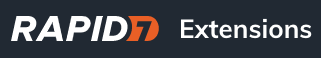# Weekly Vulnerability Counts Per Asset

I haven’t seen any examples using “fact_asset_date” so I thought I would share this query to report on total vulnerabilites, total criticals, total exploits, total risk score, etc. on a per asset basis reported in weekly intervals.

The table “fact_all_date” would be way faster, but apparently it either no longer exists or is not available in query search.

SELECT
week_of,
asset_count,
sum_vuln/asset_count AS “Vulns/Asset”,
sum_crit/asset_count AS “Crits/Asset”,
sum_exp/asset_count AS “Exp/Asset”,
sum_exp_mal/asset_count AS “Exp+Mal/Asset”,
sum_risk/asset_count AS “Total_Risk/Asset”
FROM (
SELECT
fact_asset_date.day AS week_of,
COUNT(DISTINCT asset_id) AS asset_count,
SUM(vulnerabilities) AS sum_vuln,
SUM(critical_vulnerabilities) AS sum_crit,
SUM(vulnerabilities_with_exploit) AS sum_exp,
SUM(vulnerabilities_with_exploit+vulnerabilities_with_malware_kit) AS sum_exp_mal,
SUM(riskscore) AS sum_risk
FROM fact_asset_date(‘2021-06-01’, CURRENT_DATE, INTERVAL ‘1 week’)
GROUP BY fact_asset_date.day